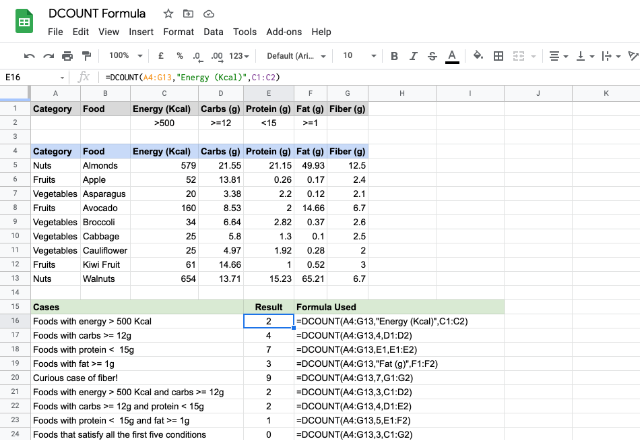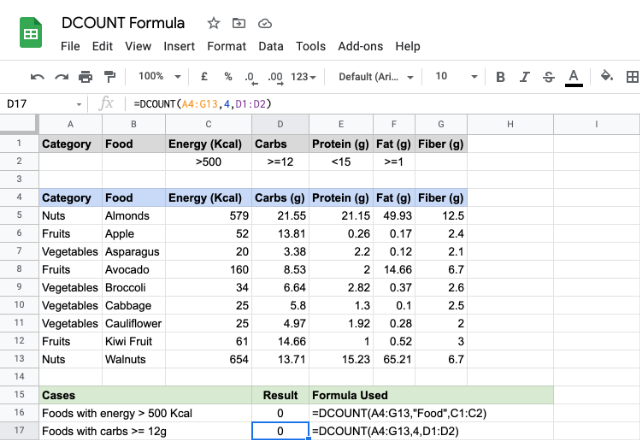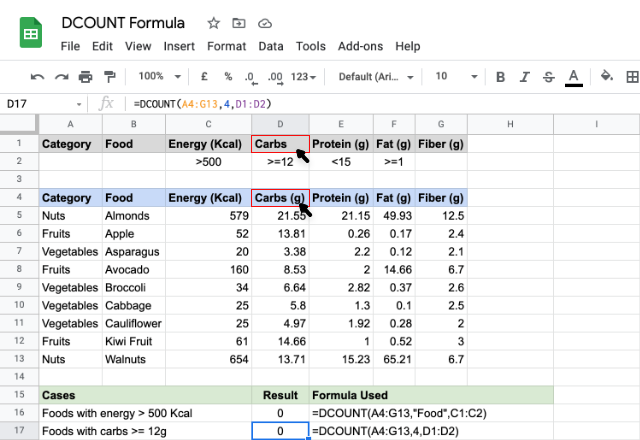# How to use the DCOUNT formula in Google SheetsThe DCOUNT formula in Google Sheets is almost similar to that of the COUNT formula, but with a distinction. It gives us the count of numeric values available in a table like range, that meet a specified criteria. This is analogous to an SQL database count query. The ‘D’ in the DCOUNT stands for ‘Database’, therefore, we can call this a Database COUNT formula.

### Syntax

DCOUNT(database, field, criteria)

• database – is the reference to a structured data range that consists of labels, for each column, in the first row.
• field – indicates the column on which the DCOUNT formula should count the numeric values. This can be a text or a column index too.
• criteria – this is a reference to a range that consists of criteria that the formula uses to filter the database values before counting.

### Usage: DCOUNT Formula

Let us try our hands with a few examples on a sample data set picked up from this website.We see that the data set is in a labeled tabular form spanning across cells A4 through to G13. And the criteria is keyed in the cells A1 through to G2.

Please note from the cases illustrated above that we can specify one or more criteria to filter the counting process. For example, the case in row # 8 counts the numeric values in the “Fat (g)” column, subject to the criteria in the cell F2. Whereas the case in row # 10 counts numeric values from “Energy (Kcal)” column, subject to the criteria within the cells C2 and D2. The last case on row # 13 includes all the criteria from C2 through to G2, and apparently there is no data that satisfies all of these conditions, hence the result 0.

The case on the row # 9 is an interesting one! We didn’t specify anything in the cell G2, yet, we gave that reference to the DCOUNT formula. The result is 9, because it counted everything in the “Fiber (g)” column as there is no criteria to filter out the rows.

##### Unwarranted Cases

Now, let us talk about pitfalls. Here is the first one, which we might already be aware of. Please consider the first case in the snapshot below.Here, we tried counting non numeric values! Just like the COUNT formula, DCOUNT formula counts only numbers. Therefore, it returned ‘0’ as output.

The second is a not so obvious as the first one. Seemingly there’s nothing wrong with the formula this time. But the problem lies within the labels. Apparently, a missing or a mismatched label doesn’t help the DCOUNT formula as its working hinges on the field names that the second parameter takes. Since the labels didn’t match, it returned the count as zero.### DCOUNT formula

And there you go! Use the DCOUNT formula in Google Sheets to count the numeric values available in a table like range, that meet a specified criteria.

If you’d like to learn more about the various formulas of Google Sheets, why not take a look at our blog post on the DCOUNTA formula in Google Sheets.

Alternatively, check out related blog posts below!